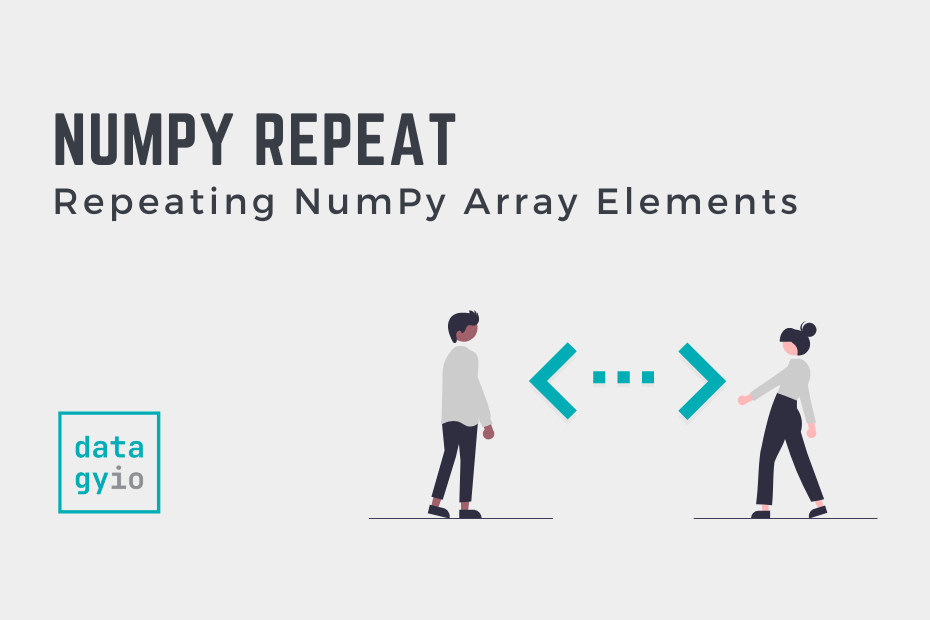# NumPy Repeat Function: Repeating NumPy ArraysIn this tutorial, you’ll learn how to use the NumPy repeat() function. The `np.repeat()` function is used to repeat arrays and provides significant opportunity to customize how the arrays are repeated. Being able to work with NumPy arrays is an important skill for data analysis, data science, and deep learning.

By the end of this tutorial, you’ll have learned:

• How the NumPy repeat function works
• How to repeat NumPy arrays row-wise and column-wise
• How to repeat multi-dimensional data in NumPy

## Understanding the NumPy repeat Function

Before diving into how the NumPy repeat function work, it’s important to understand the syntax of the function. The code block below shows the syntax of the function and all of its available parameters:

``````# Understanding the Syntax of np.repeat()
np.repeat(
a,
repeats,
axis=None
)``````

We can see from the code block above that the function has three different parameters. Let’s take a look what each of these accomplish:

• `a=` accepts the input array
• `repeats=` identifies the number of repetitions for each element
• `axis=` identifies the axis along which to repeat values. If `None`, uses the flattened array as input and returns a flattened array.

## Repeating a Single Dimensional NumPy Array

By applying the NumPy repeat() function to a one-dimensional array, the values in that array are repeated. What may surprise you is that the repetition occurs element-wise. To better understand what this looks like, let’s take a look at an example:

``````# Repeating a 1-D Array using np.repeat()
import numpy as np

arr = np.array([1, 2, 3])
print(np.repeat(arr, 2))

# Returns:
# [1 1 2 2 3 3]``````

In the example above, we pass a one-dimensional NumPy array into the `repeat()` function. We also instruct the function to repeat each element twice.

Rather than returning the entire array following one another, each element is repeated in sequence. In the following section, you’ll learn how to repea elements in a two-dimensional array.

## Repeating a Two-Dimensional NumPy Array

In this section, you’ll learn how to repeat elements of a two-dimensional array. In the previous section, you learned that items are repeated element-wise, rather than array-wise.

In this section, you’ll learn that, by default, the function will return a flattened array. This means that the elements are first reshaped into a one-dimensional array and then repeated.

Let’s take a look at an example to better understand this:

``````# Repeating a 2-D NumPy Array with np.repeat()
import numpy as np

arr = np.array([[1,2],[3,4]])
print(np.repeat(arr, 2))

# Returns:
# [1 1 2 2 3 3 4 4]``````

We can see in the example above that the values are first flattened and then repeated. This is because, by default, the `axis` parameter is set to `None`.

In the following section, you’ll learn how to customize this behaviour to repeat items colume-wise.

## Repeating a NumPy Array Row-Wise

In many cases, you may not want to repeat a multi-dimensional array in a flattened version of itself. You can customize this behaviour by modifying the `axis` parameter.

In NumPy, the 0th axis represents the rows. Let’s pass in `0` into the `axis` parameter and see how this modifies the behaviour:

``````# Repeating Items Column-wise in NumPy
import numpy as np

arr = np.array([[1,2],[3,4]])
print(np.repeat(arr, 2, axis=0))

# Returns:
# [[1 2]
#  [1 2]
#  [3 4]
#  [3 4]]``````

In the example above, we passed in the same two-dimensional array and repeated it twice along the zeroth axis. In this case, each “row” is repeated.

In the following section, you’ll learn how to repeat items column-wise.

## Repeating a NumPy Array Column-Wise

In the previous section, you learned how to repeat the “rows” of a NumPy array using the `np.repeat()` function. In this section, you’ll learn how to repeat items column-wise, meaning that each “column” is repeated.

Let’s see how we can modify the behaviour of the `axis` parameter to accomplish this:

``````# Repeating Items Row-Wise in NumPy
import numpy as np

arr = np.array([[1,2],[3,4]])
print(np.repeat(arr, 2, axis=1))

# Returns:
# [[1 1 2 2]
#  [3 3 4 4]]``````

We can see here that each “column” of the array is repeated.

## Conclusion

In this tutorial, you learned how to use the NumPy repeat function to repeat items in a NumPy array. The function allows you to repeat items in an array element-wise, which may surprise users of the function. You first learned what parameters are available in the function and how to work with single-dimensional arrays.

Then, you learned how to use the function with two-dimensional arrays. The function, by default, flattens the array and repeats the elements. By modifying the function’s behaviour, the function can be used to repeat elements row-wise or column-wise.